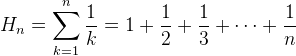Computes the given harmonic number.

Namespace:  Meta.Numerics.Functions
Assembly:  Meta.Numerics (in Meta.Numerics.dll) Version: 4.1.4Syntax
```public static double HarmonicNumber(
int n
)```

#### Parameters

n
Type: SystemInt32
The index of the harmonic number to compute, which must be non-negative.

#### Return Value

Type: Double
The harmonic number Hn.Exceptions
ExceptionCondition
ArgumentOutOfRangeExceptionn is negative.
ArgumentOutOfRangeExceptionn is negative.Remarks

Hn is the nth partial sum of the harmonic series.Since the harmonic series diverges, Hn grows without bound as n increases, but it does so extremely slowly, approximately as log(n).See Also